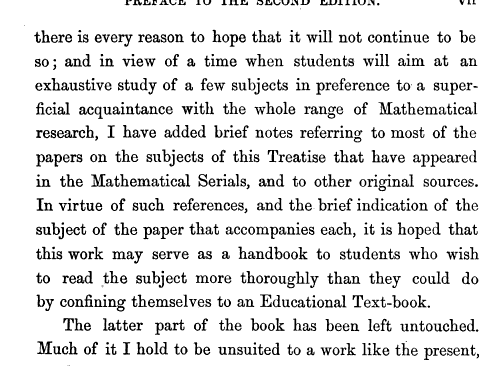## Thursday, January 1, 2009

### A Treatise on the Calculus of Finite DifferencesBoole, George. "A Treatise on the Calculus of Finite Differences", Macmillan and Co., 1872.

Even though it was written in 1860, this book probably even more relevant today than it was then. Why? Because it provides the mathematical basis for solving higher and higher resolution partial differential equations with faster and faster computers for the complex domains of modern engineering problems. The faster, more accurate and more efficient solution to these types of problems provides the foundation for improving quality of life for everyone.

I like his definition of the calculus of finite differences, it says so much, succinctly:
The Calculus of Finite Differences may be strictly defined as the science which is occupied about the ratios of the simultaneous increments of quantities mutually dependant.

This book has to be understood as a second volume to accompany Boole's first book on Differential Equations. He stresses the importance of the relationship between the two in his preface to TCFD. The discussion of the distinction between a calculus of limits and the calculus of differences highlights this connection.

Like most old math or science books, it is the discussion of important applications that feels dated. The ideas are timeless, but the important applications change with the problems and the technology of the time.

Another timeless idea that bears repeating around all practitioners of the numerical art (from Chapter 8):

... we shall very often need to use the method of Finite Differences for the purpose of shortening numerical calculation, and here the mere knowledge that the series obtained are convergent will not suffice; we must also know the degree of approximation.

To render our results trustworthy and useful we must find the limits of the error produced by taking a given number of terms of the expansion instead of calculating the exact value of the function that gave rise thereto.